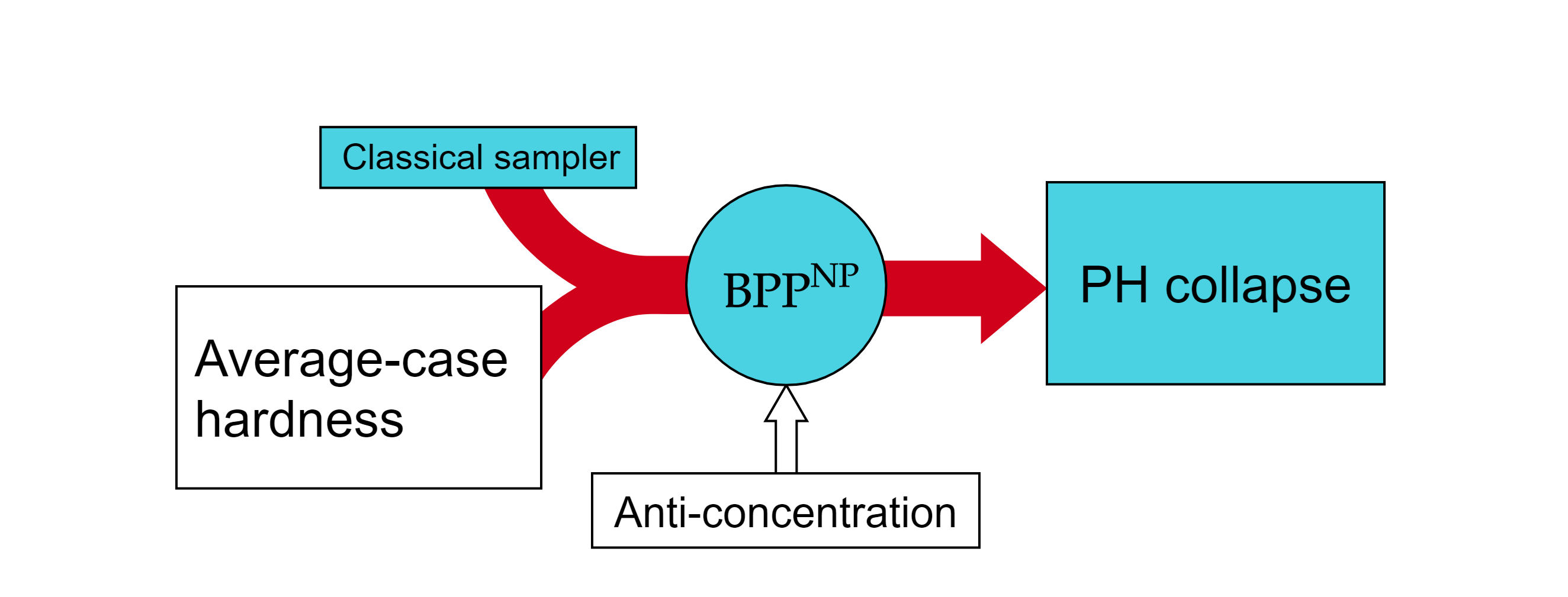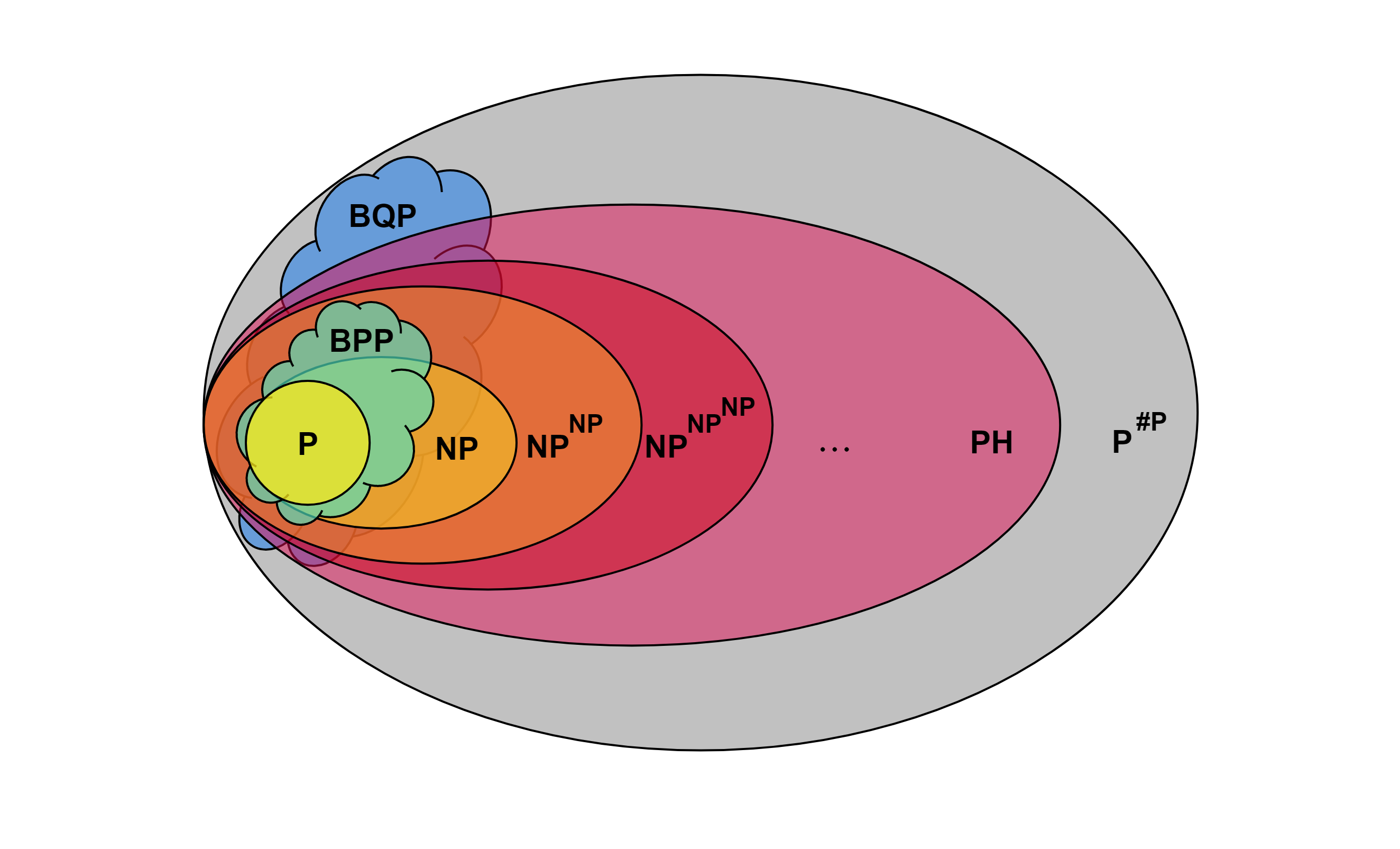# Ingredients for quantum supremacy [Draft]

Epistemic status: Summarizing research literature

Is there a task in which quantum computers can achieve beyond what any classical algorithm can do, even those yet undiscovered? The search for a rigorous, complexity-theoretic proof of the so-called quantum (computational) supremacy 1 goes back to the 2002 preprint by Terhal and DiVincenzo. Subsequent theoretical works on quantum supremacy all rule out classical simulations of certain quantum systems by arguing that if such simulations are possible, then there will be a devastating consequence, “collapse of the polynomial hierarchy (PH)”, which is deemed extremely unlikely by computer scientists. Such an argument is not known for factoring; if tomorrow someone figures out a fast way to factor integers on a classical computer 2, we will have to switch to a different encryption scheme but that’s about it. The concept of reality as we know will remain unchallenged, while a collapse of the polynomial hierarchy will bring us closer to the world in which P=NP—from Russell Impagliazzo’s A Personal View of Average-Case Complexity,

Algorithmica is the world where $\cc{P=NP}$ or its moral equivalent (e.g., $\cc{NP\subseteq BPP}$). To be more concrete, let’s define Algorithmica as the world where there exists a simple and magical linear time algorithm for the SAT problem. […] this world is a computational utopia. We would be able to automate various tasks that currently require significant creativity: engineering, programming, mathematics, and perhaps even writing, composing, and painting. On the other hand, this algorithm could also be used to break cryptographic schemes, and hence almost all of the cryptographic applications currently used will disappear.

There are plenty of public misconceptions about quantum supremacy, as witnessed from the Google’s announcement. But in this blog post I will talk about misconceptions among scientists who are not familiar with computational complexity.

How do we go about proving the collapse of PH?

If FP is the class of problems that can be solved by a flying pig, and you have a strong reason to believe that it has a certain relationship with another complexity class, then a mathematical statement that implies the opposite of such relationship would be thought of as unlikely regardless of whether a flying pig exists or not.

# NP oracle and the polynomial hierarchy

### What does an NP oracle actually do?

An oracle for a problem $O$ is a magical black box that can answer the question $O$ with a single querying. Generally having access to an oracle boosts the power of a computational model. For instance, one can have an oracle for an undecidable problem; there is no Turing machine that can decide whether an arbitrary Turing machine halts or not, but an oracle for the halting problem (upon inputting a Turing machine $M$) would just give the one-bit answer HALT or NOT HALT in one step.

Similarly, one can have an oracle for an NP-complete problem, 3SAT for concreteness. Every Boolean formula can be expressed in the conjunctive normal form (CNF) $\vee_j \left(\wedge_k u_{jk}\right)$ where each $u_{jk}$ is a Boolean variable, $j$ labels the clauses, and $k$ labels the literals. The problem kSAT asks if a given Boolean formula wherein each clause has $k$ literals is satisfiable i.e. the value of each $u_{jk}$ can be set so that the formula evaluates to TRUE. NP is the class of problems where there is a short certificate for every YES instance. For example in 3SAT, if a Boolean formula is satisfiable, I can prove it by giving you a set of satisfying arguments. SAT and 3SAT are NP-complete, while 2SAT is in P.

But note the asymmetry; there is no obvious short proof that I can show to convince you that a Boolean formula is unsatisfiable without trying all combinations of the Boolean variables. A class of problems such that there is a short proof for every NO instance is called coNP. Factoring is special in that both YES and NO instances can be checked quickly, so it is in $\mathrm{NP \cap coNP}$ and is not believed to be NP-complete.

For the above reason, $\mathrm{P^{NP}}$ is believed to be larger than NP (containing both NP and coNP) because when I ask the oracle “is this Boolean formula satisfiable?”, the oracle can give the answer NO, which seems to be beyond the capability of an NP machine. Similarly, $\mathrm{NP^{NP}}$ is believed to be larger than NP. Hence we define the polynomial hierarchy to be the infinite tower $\mathrm{NP^{NP^{NP^{\cdots}}}}$, each level $\Sigma_{k+1} = \Sigma_k^{\mathrm{NP}}$ appears to be more computationally powerful than the one below. ($\Sigma_0 \coloneqq \mathrm{P}$ and $\Sigma_1 \coloneqq \mathrm{NP}$.)

Random quantum circuits

Now switching from a very general exposition to a technical question that I’m interested in: what are the ingredients for proofs of quantum supremacy, particularly in a random quantum circuit architecture? In this setting, there is an ensemble $\mathcal{E}$ of quantum circuits $U$and we want to show that classically “simulating” the outcome probabilities $p_x = |\av{x|U|0}|^2$ for some $x \in \{0,1\}^{2^n}$ leads to $\cc{PH}$ collapse and therefore such a simulation is likely impossible. There are two major “axes” of possible kinds of simulations.

1. By “simulate”, we may want to estimate $p_x$ up to a multiplicative precision 3 $|p_x - q_x| \le \eta p_x,$ or we just want to estimate $p_x$ up to an additive precision $|p_x - q_x| \le \epsilon.$

2. We may want to simulate all quantum circuits in $\mathcal{E}$ or just some fraction of them i.e. on average.

To get the strongest result, we would like to assume weakest notion of simulation,

a result that says that additive approximation of $p_x$ by a classical machine is hard on average. The main goal of this blog post is to figure out how much we presently can and can’t say along this line.

Bremner’10|(Multiplicative)
Aaronson’11
Bremner’10|Postselection| |Average|Aaronson’11
Bouland’18|Conjectured
Bremner’16
Boixo’18|Stockmeyer
Random circuits anticoncentrate|

# Anatomy of average-case argument

Most proposals of quantum supremacy relate sampling from quantum circuits to a $\cc{\#P}$-complete problem. $\cc{\#P}$ is a class of problems that count the number of solutions to an $\cc{NP}$ problem (thus at least as hard as $\cc{NP}$). The suffix “-complete” means that the problem is at least as hard as any problem in the complexity class and is itself in the class. Examples of $\cc{\#P}$-complete problem are computing the permanent 4 of a matrix and counting perfect matchings of a graph. For physicists, bosons are “permanental” while fermions are “determinantal”. That’s why Boson-Sampling is hard while the analogous task of simulating fermion number states is easy.

It is a fairly common misconception that these hard problems can be efficiently solved by a quantum computer (by simulating Boson-Sampling for instance). So let me state this clearly: quantum computers are not believed to be able to solve $\cc{NP}$-complete problems efficiently, let alone $\cc{\#P}$-complete problems. The arguments for quantum supremacy are more subtle and rely on an unphysical computation (assuming $\cc{P \neq NP}$) known as Stockmeyer’s counting. In particular, a classical machine that can sample from $p_x$ with additive precision will let you solve a $\cc{\#P}$-complete problem on average only if you also have an $\cc{NP}$ oracle.The arguments for quantum supremacy are more subtle and rely on an unphysical process known as Stockmeyer’s counting that, given a classical machine that outputs $q_x$, there is a $\cc{BPP^{NP}}$ machine that produces a multiplicative estimate $\tilde{q}_x$ to $q_x$. Aaronson and Arkhipov’s insight was that sometimes $\tilde{q}_x$ is also a multiplicative estimate to the probabilities $p_x$ from a quantum device, to which the output $q_x$ of the classical device is an additive estimate. In other words, Stockmeyer’s counting can sometimes improves an additive estimate to a multiplicative one. Therefore, additive approximation to $p_x$ doesn’t let you solve a $\cc{\#P}$-complete problem in polynomial time unless you also have an (unphysical) $\cc{NP}$ oracle.

## Good multiplicative approximation in $\cc{BPP^{NP}}$

also repeated for random quantum circuits in Appendix G of

Suppose that there exists a classical sampler that gives an additive estimate $q_x$ of $p_x$: $\sum_{x\in\{0,1\}^{2^n}} |p_x - q_x| \le \epsilon.$ By Stockmeyer’s counting, $q_x$ can be approximated with multiplicative precision by a $\mathrm{BPP^{NP}}$ machine $|q_x - \tilde{q}_x| \le \frac{q_x}{\mathrm{poly}(n)}.$ Let’s combine these two facts together. \begin{align} |p_x-\tilde{q}_x| &\le |p_x-q_x| + |q_x - \tilde{q}_x| &\text{Triangle inequality}\\ &\le |p_x-q_x| + \frac{q_x}{\mathrm{poly}(n)} &\text{Stockmeyer's counting}\\ &\le |p_x-q_x| + \frac{|p_x - q_x| + p_x}{\mathrm{poly}(n)} &\text{Triangle inequality}\\ &= \frac{p_x}{\textrm{Poly}(n)} + |p_x-q_x|\left(1 + \frac{1}{\textrm{poly}(n)}\right) \end{align} If $|p_x-q_x|$ can be bounded above by $p_x$, then $\tilde{q}_x$ is a multiplicative approximation to $p_x$ that we want. This is done in two steps:

1. Use Markov’s inequality \begin{align} \mathrm{Pr} \left[ |p_x-q_x| \ge \frac{\epsilon}{2^n\delta} \right] \le \frac{\mathbb{E}(|p_x-q_x|)2^n\delta}{\epsilon} \le \delta \iff \mathrm{Pr} \left[ |p_x-q_x| \le \frac{\epsilon}{2^n\delta} \right] \ge 1-\delta \end{align} to bound $|p_x-q_x|$ by a constant for $1-\delta$ fraction of quantum circuits.

2. Invoke “anti-concentration” of probabilities (conjectured for BosonSampling, proved for random quantum circuits and more) \begin{align} \mathrm{Pr} \left[ p_x > \frac{\epsilon}{2^n\delta\eta} \right] &\ge \gamma, \end{align} to bound the constant by $p_x$, where $\eta$ is some small constant.

Now $(1- \delta)\cap\gamma$ fraction of quantum circuits will satisfy \begin{align} |p_x-\tilde{q}_x| &\le p_x\left(\eta + \frac{1}{\mathrm{poly}(n)}\right). \end{align} For $(1- \delta)\cap\gamma$ to be non-empty, we need to pick $\delta$ such that $(1- \delta)\cup\gamma > 1$.

If $p_z$ is $\cc{\#P}$-hard to compute for this fraction of quantum circuits, then we have shown that $\cc{P^{\#P}}\subset\cc{BPP^{NP}}$ which is contained in the third level of $\cc{PH}$ by the Sipser–Gács–Lautemann theorem on the one hand. On the other hand, $\cc{PH}\subset\cc{P^{\#P}}$ by Toda’s theorem, so $\cc{PH}$ collapses to the third level.Fun fact: exactly computing probabilities, even for a subclass of quantum circuits that does only $\cc{BPP}$ computation (a layer of Hadamard’s to simulate coin flips, followed by diagonal gates) is $\cc{\#P}$-complete 5. In particular, you can create the state $\propto \sum_x \ket{x}\ket{f(x)}$ for any Boolean function $f$. Counting the number $\#f$ of the inputs such that $f(x)=0$ is by definition $\cc{\#P}$-complete. Exactly computing the outcome probability $\mathrm{Pr}(0) = \#f/2^n$ when measuring the second qubit is therefore $\cc{\#P}$-complete. since it’s a $\cc{BPP}$ computation, obviously you can just sample the probability. What prevents PH collapse from this result?

# Anti-concentration

## By unitary 2-designs

Paley-Zygmund inequality

Counterexample: Clifford circuits with $\ket{0}^{\otimes n}$ input state.

# Average-case hardness for random quantum circuits

1. coined by John Preskill and disliked by many because of the word’s racial connotation [scirate] [Nature correspondence]

2. Henry Cohn’s “Factoring may be easier than you think

3. Multiplicative approximation is much stronger than additive one; if $p_x = 0$, then a multiplicative estimate $q_x$ must exactly equal $p_x$.

4. The permanent is like the determinant but without the minus signs.

5. Multiplicative approximation is much stronger than additive one; if $p_x = 0$, then a multiplicative estimate $q_x$ must exactly equal $p_x$.

Archieves by date, by category, by tag.